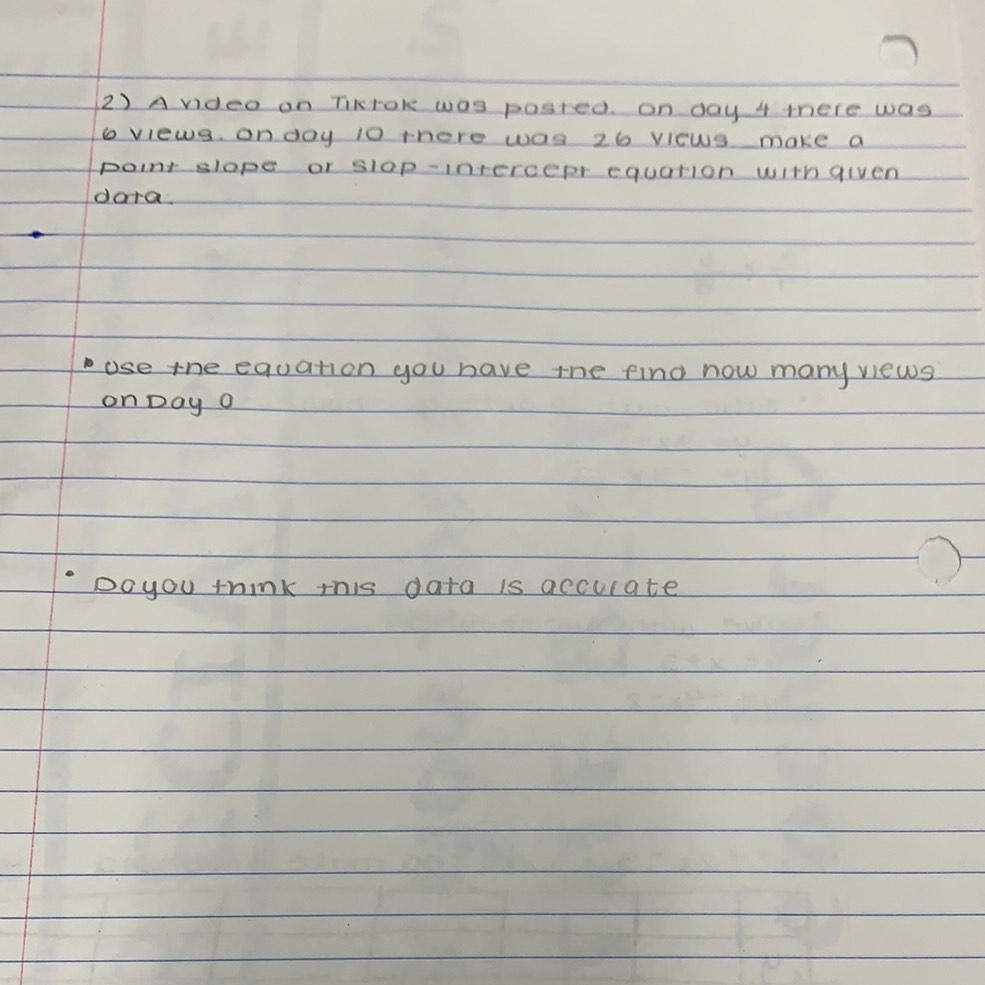Question:

# 1. A video on Tiktok was posted. on day 4 there was 6 views on day 10 there was 26 views make a point slope or slap-intercept equation with given data. 2. use the equation you have the find how many1. A video on Tiktok was posted. on day 4 there was 6 views on day 10 there was 26 views make a point slope or slap-intercept equation with given data. 2. use the equation you have the find how many views on day 0. 3. Do you think this data is accurate.